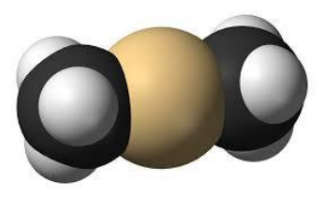# Problem: Dimethylcadimum, Cd(CH3)2, is considered to be one of the most dangerous chemicals on Earth. It is volatile, poisonous, carcinogenic and explosive. What is the mass percent of cadmium in dimethylcadmium?A. 88.2%B. 16.9%C. 78.9%D. 4.2%E. 51.0%

🤓 Based on our data, we think this question is relevant for Professor Morgan's class at ASU.

###### FREE Expert Solution

Figure out the molar mass of the Cd(CH3)2 and divide it by the atomic mass of Cd to find the mass percent of Cd in the compound where:

Step 1. Determine the molar mass of Cd(CH3)2

Step 2. Divide the atomic mass of Cd over the molar mass of Cd(CH3)2

Step 1. Determine the molar mass of Cd(CH3)2###### Problem Details

Dimethylcadimum, Cd(CH3)2, is considered to be one of the most dangerous chemicals on Earth. It is volatile, poisonous, carcinogenic and explosive. What is the mass percent of cadmium in dimethylcadmium?A. 88.2%
B. 16.9%
C. 78.9%
D. 4.2%
E. 51.0%